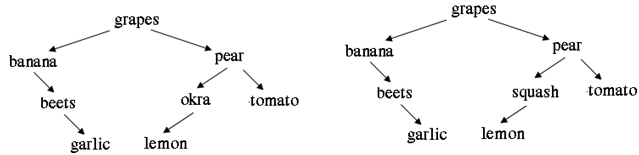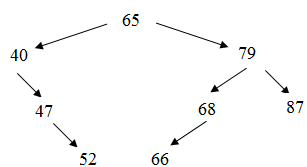## Recursive function, Data Structure & Algorithms

Assignment Help:

The location of a node in a binary search tree is defined as a string such as LLRRL, which represents the node that you find by starting at the root, and traversing Left, traverse Left, traverse Right, traverse Right, and traverse Left.   Write a function to print a path to some target 'x' based on the direction from the root to the target.  For example, in the tree above, the path from 65 to 52 is LRR. Hint: use a stack to store the path.Write a recursive algorithm to count the number of right children in a binary search tree.

Write the method levelCount whose header is given below. Method levelCount returns the number of nodes on the specified level.#### Complete trees, This is a k-ary position tree wherein all levels are filled...

This is a k-ary position tree wherein all levels are filled from left to right. There are a number of specialized trees. They are binary trees, AVL-trees, binary search trees, 2

#### Travelling sales person, explanation with algorithm

explanation with algorithm

#### Bubble sort, #question bubble sort..

#question bubble sort..

#### Graph with n vertices will absolutely have a parallel edge, A graph with n ...

A graph with n vertices will absolutely have a parallel edge or self loop if the total number of edges is greater than n-1

#### Explain the term group support system, (a) Explain the term Group Support S...

(a) Explain the term Group Support System and elaborate on how it can improve groupwork. (b) Briefly explain three advantages of simulation. (c) Explain with the help of a

#### Explain expert system, 1. What is an expert system and where are they need...

1. What is an expert system and where are they needed? 2. What are the major issues involved in building an expert system?

#### Procedure to delete all terminal nodes of the tree, Q. Let a binary tree 'T...

Q. Let a binary tree 'T' be in memory. Write a procedure to delete all terminal nodes of the tree.       A n s . fun ction to Delete Terminal Nodes from Binary Tree

#### Insertion of an element in a linear array, To delete an element in the list...

To delete an element in the list at the end, we can delete it without any difficult. But, assume if we desire to delete the element at the straining or middle of the list, then, we

#### Applications of linear and binary search, The searching method are applicab...

The searching method are applicable to a number of places in current's world, may it be Internet, search engines, text pattern matching, on line enquiry, finding a record from data

#### Undirected graph and adjacency matrix, Q. Consider the specification writte...

Q. Consider the specification written below of a graph G V(G ) = {1,2,3,4} E(G ) = {(1,2), (1,3), (3,3), (3,4), (4,1)} (i)        Draw the undirected graph. (